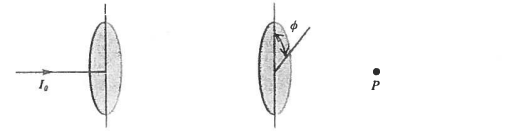# Problem: Light with intensity I0 = 300 W/m2 passes through two ideal polarizing filters, which are oriented as shown in the figure. The angle of the first polarizer is fixed, while the angle Φ of the second filter is adjustable. a) If the initial light is unpolarized, what value of  Φ will make the intensity at point P equal to 100 W/m2? Φ = _______________   b) Assuming the same conditions as in part (a), what are the maximum electric and magnetic field values at point P? (ε0 = 8.854 x 10-12 C2 / (N•m2)) Emax = _______________ Bmax = _______________   c) Now assume the inital light is linearly polarized in the same direction as the polarizing axis of the first polarizer. If Φ is fixed at the angle found in part (a), what will the intensity at point  P be? I = _______________

🤓 Based on our data, we think this question is relevant for Professor Ford's class at TAMU.

###### Problem Details

Light with intensity I= 300 W/m2 passes through two ideal polarizing filters, which are oriented as shown in the figure. The angle of the first polarizer is fixed, while the angle Φ of the second filter is adjustable.

a) If the initial light is unpolarized, what value of  Φ will make the intensity at point P equal to 100 W/m2?

Φ = _______________

b) Assuming the same conditions as in part (a), what are the maximum electric and magnetic field values at point P? (ε0 = 8.854 x 10-12 C/ (N•m2))

Emax_______________

Bmax_______________

c) Now assume the inital light is linearly polarized in the same direction as the polarizing axis of the first polarizer. If Φ is fixed at the angle found in part (a), what will the intensity at point  P be?

I _______________